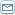By: Florian Ion Tiberiu PetrescuABOUT ENERGY Florian Ion TIBERIU-PETRESCU? The paper presents, shortly, some relations which may deduce the total energy of a corpuscle, W, the kinematical energy of this particle, E, and the energy DE that increases the corpuscle mass, from the rest mass, m0, to mass m. Generally, one considers the DW energy like the exactly kinematical energy and E energy like an approximately kinematical energy. In this paper one presents an original method to deduce the kinematical energy E, starting from kinematical Force (Lagrange Force). The author wants (in this mode) establish the E energy of a corpuscle like the exactly kinematical energy. If E is the really kinematical energy of a corpuscle, what’s the DW energy? DW represents a sum between two energy: E (the kinematical energy) and DE (the energy which increases the mass of a corpuscle from the rest mass, m0, to m mass, when the particle speeds from v=0 to v). ? Keywords: Particle, corpuscle, electron, mass, rest mass, Lagrange force, quantum, energy, total energy-W, kinematical energy-E, increase mass energy-DE, a sum energy-DW=E+DE.? ? See the paper from my webpage:http://www.google.com/base/search?authorid=1450191???? ? One integrates the expression (7) and one obtains the form (8), for the total energy W (when m=0, W=0, and? the integration constant=0).?? ??? Relation (8)represents the total energy of a corpuscle, obtained without relativity theory (8). With the Lorentz expression (5) the relation (8) takes the forms (9).? One note with DW, the difference between the energy W (for a velocity v and a mass m) and the rest energy W0 (when the velocity v=0 and the corpuscle mass become the rest mass, m0).? The kinematical force (Lagrange Force) can be written in the (3) form.? See the difference between this force, (kinematical force) and the general force (relation 2).? The kinematical elemental energy of a corpuscle can be written in form (4’).? Integrating the relation (4’) the kinematical energy, E, takes the form (11), (where the integration constant=0, when E=0 and m=0).? And with Lorentz expression (5), the kinematical energy, E, take the form (12).? One can see easily, that DW energy is greater than E energy (kinematical energy), (relation 13).? DW energy is a sum between energy E and DE. We know DW and E, and one calculates DE like a difference between DW and E.? The relation (14), DE, represents the difference between the DW (a difference of Einstein energy) and the kinematical energy, E (Lagrange Energy). This difference, DE, may be the energy which increase the corpuscle mass, from the rest mass, m0, to mass m, when the particle speeds from rest velocity, v=0, to v. ?-for the velocity v=0, DE=0, and DW=E;?-for the velocity v=c, DE=E, and? DW=2.E;?? Conclusions? When DW become the double of kinematical energy, E, and DE become E, the speeded corpuscle take the constant velocity of light, c, and become a particle without electric load.? ?See the paper from one of these sites:http://iondynamics.blogspot.comhttp://dynamicsp.blogspot.comhttp://atomicmodel.blogspot.com